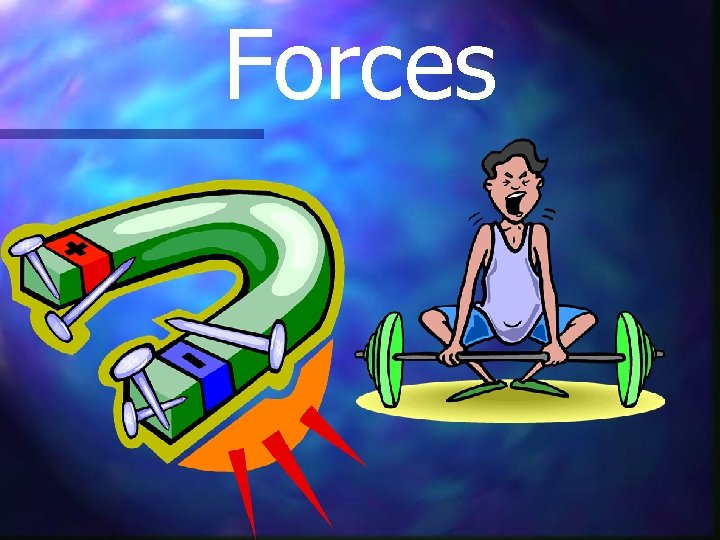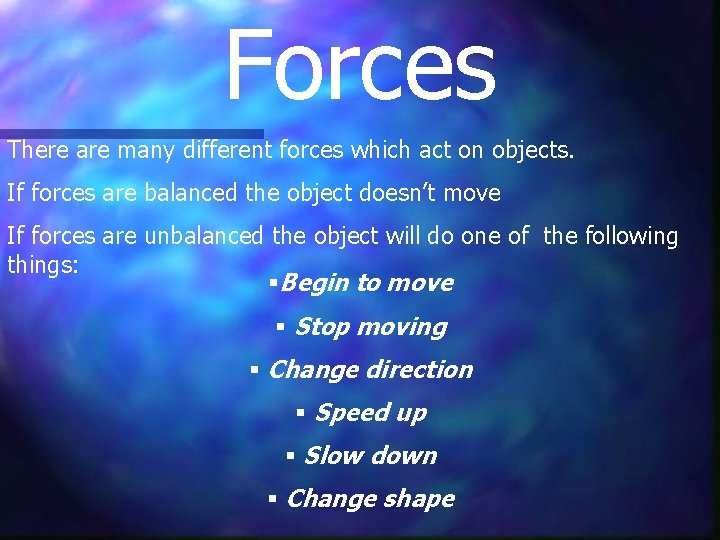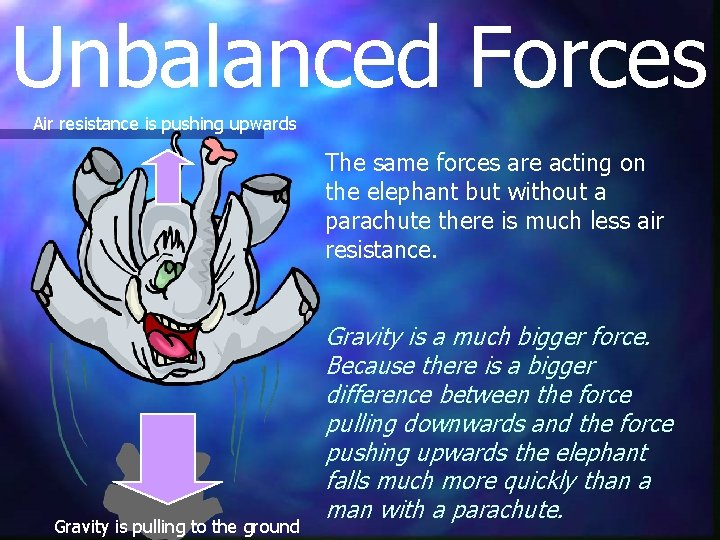Forces Forces There are many different forces which

• Slides: 8ForcesForces There are many different forces which act on objects. If forces are balanced the object doesn’t move If forces are unbalanced the object will do one of the following things: §Begin to move § Stop moving § Change direction § Speed up § Slow down § Change shapeBalanced Forces The ship is floating on the water, the forces are balanced. Which forces are acting on the ship? Gravity Because Gravity and Upthrust are equal the ship stays afloat. (Water’s) UpthrustBalanced Forces The vase is resting on the table. It is not moving, therefore the forces must be balanced. Which forces are acting on the vase? Have a look around the classroom and name some of the balanced forces in action on different objects. Gravity Table’s UpthrustUnbalanced Forces Air resistance is pushing upwards The man and the parachute are slowly falling to the ground. Which forces are acting on the parachute? Which force is bigger? How can you tell? Gravity is the bigger force. We can tell this because the direction of movement of the man and the parachute is the same as the direction of the force of gravity. Gravity is pulling the man to the groundUnbalanced Forces Air resistance is pushing upwards The same forces are acting on the elephant but without a parachute there is much less air resistance. Gravity is pulling to the ground Gravity is a much bigger force. Because there is a bigger difference between the force pulling downwards and the force pushing upwards the elephant falls much more quickly than a man with a parachute.Unbalanced Forces The paperclip is jumping up to the magnet. The magnetic force is pulling the paperclip upwards Gravity is pulling the paperclip to the ground Which forces are acting on the magnet? Which is the greater force? The magnetic force is greater than the force of gravity therefore the paperclip moves in the direction of the biggest force.Forces Each team is exerting a force – what happens if the pulling force produced by each team is equal? What would happen if the team on the left were able to produce a bigger pulling force than the team on the right?